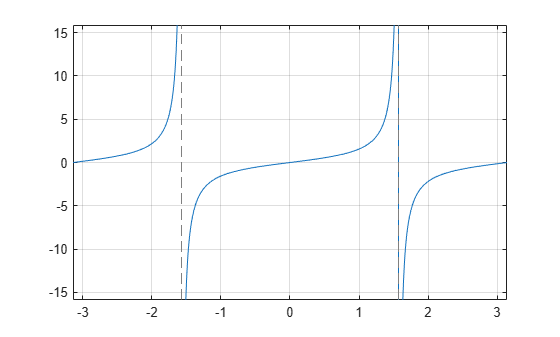# tan

Symbolic tangent function

## Syntax

``tan(X)``

## Description

example

``tan(X)` returns the tangent function of `X`.`

## Examples

### Tangent Function for Numeric and Symbolic Arguments

Depending on its arguments, `tan` returns floating-point or exact symbolic results.

Compute the tangent function for these numbers. Because these numbers are not symbolic objects, `tan` returns floating-point results.

`A = tan([-2, -pi, pi/6, 5*pi/7, 11])`
```A = 2.1850 0.0000 0.5774 -1.2540 -225.9508```

Compute the tangent function for the numbers converted to symbolic objects. For many symbolic (exact) numbers, `tan` returns unresolved symbolic calls.

`symA = tan(sym([-2, -pi, pi/6, 5*pi/7, 11]))`
```symA = [ -tan(2), 0, 3^(1/2)/3, -tan((2*pi)/7), tan(11)]```

Use `vpa` to approximate symbolic results with floating-point numbers:

`vpa(symA)`
```ans = [ 2.1850398632615189916433061023137,... 0,... 0.57735026918962576450914878050196,... -1.2539603376627038375709109783365,... -225.95084645419514202579548320345]```

### Plot Tangent Function

Plot the tangent function on the interval from $-\pi$ to $\pi$.

```syms x fplot(tan(x),[-pi pi]) grid on```### Handle Expressions Containing Tangent Function

Many functions, such as `diff`, `int`, `taylor`, and `rewrite`, can handle expressions containing `tan`.

Find the first and second derivatives of the tangent function:

```syms x diff(tan(x), x) diff(tan(x), x, x)```
```ans = tan(x)^2 + 1 ans = 2*tan(x)*(tan(x)^2 + 1)```

Find the indefinite integral of the tangent function:

`int(tan(x), x)`
```ans = -log(cos(x))```

Find the Taylor series expansion of `tan(x)`:

`taylor(tan(x), x)`
```ans = (2*x^5)/15 + x^3/3 + x```

Rewrite the tangent function in terms of the sine and cosine functions:

`rewrite(tan(x), 'sincos')`
```ans = sin(x)/cos(x)```

Rewrite the tangent function in terms of the exponential function:

`rewrite(tan(x), 'exp')`
```ans = -(exp(x*2i)*1i - 1i)/(exp(x*2i) + 1)```

### Evaluate Units with `tan` Function

`tan` numerically evaluates these units automatically: `radian`, `degree`, `arcmin`, `arcsec`, and `revolution`.

Show this behavior by finding the tangent of `x` degrees and `2` radians.

```u = symunit; syms x f = [x*u.degree 2*u.radian]; tanf = tan(f)```
```tanf = [ tan((pi*x)/180), tan(2)]```

You can calculate `tanf` by substituting for `x` using `subs` and then using `double` or `vpa`.

## Input Arguments

collapse all

Input, specified as a symbolic number, scalar variable, matrix variable (since R2021a), expression, or function, or as a vector or matrix of symbolic numbers, scalar variables, expressions, or functions.

collapse all

### Tangent Function

The tangent of an angle, α, defined with reference to a right angled triangle is

.The tangent of a complex argument, α, is

`$\text{tan}\left(\alpha \right)=\frac{{e}^{i\alpha }-{e}^{-i\alpha }}{i\left({e}^{i\alpha }+{e}^{-i\alpha }\right)}\text{\hspace{0.17em}}.$`

.

## Version History

Introduced before R2006a